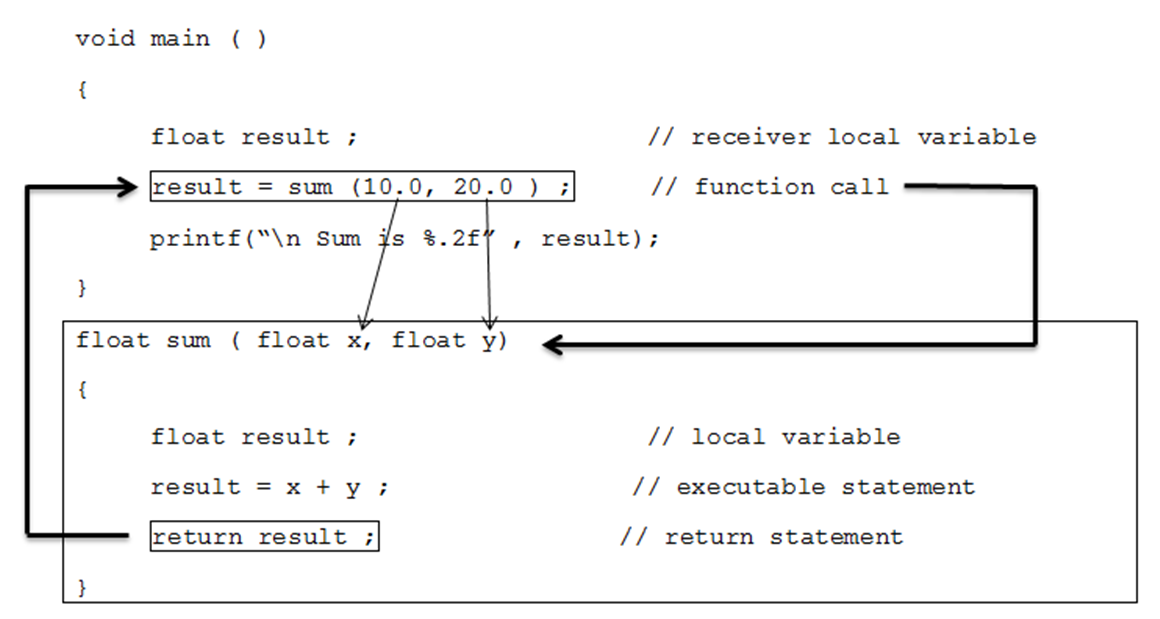• Hello everyone!. You might remember about my last post on “Introduction to Function” in which I had discussed about the what function is and what advantages it can bring. If you haven’t read my previous post yet, I strongly suggest you to read it first as this post is a supplementary for the same.
• In this post as promised I will try to explain basic elements of functions in as easy way as possible.

### Elements of Functions

• Every function has the following elements:
1. Function Declaration (Function Prototype)
2. Function Definition
3. Function Call
1. ### Function Declaration (Function prototype)

• Similar to variables, in C, every function needs to be declared before it can be used.
• Functions can be declared either globally (inside the main() ) or locally (outside the main() ) as per the program requirement. (You can read more on this at Structure of C Program)

Syntax:

``return_type function_name( argument list ) ;``
• Function prototype has the following three parts:
##### Return type :
• It specifies return type of the function that means type of the data that a function will return such as int, float, char etc.
• Function that does not return any value will have return type as void.
##### Function Name:
• It is the name of the function and it should be any meaningful name.
• It should follow all naming convention rules.
• Some examples of function names are display, show, calculate etc.
##### Argument List:
•  Argument list specifies list of all arguments required for the function to perform the desired task.
• Arguments are separated by comma. It may include both data type and argument name but normally only data type is provided.
• The following is an example of function prototype for doing sum of two float values:
###### Function Declaration Example
`float sum ( float, float ) ;  OR  float sum (float x, float y) ;`
• Where, int is a return type, sum is the name of the function and int x, int y are the argument list.
1. ### Function Definition

• Function definition contains executable block of statements.
• It can be written either before or after the main() but normally it is written after the main().

Syntax:

``````return_type function_name( argument list )
{
// local variable declaration ;

// executable statements ;

:
:

// return statement ;
}``````
• The function definition contains the following two parts:
2. Function Body

• The first line of function definition is known as function header.
• It consists of three parts: return type, function name and argument list.
1. #### Function Body:

• It consists of three parts: local variable declaration part, executable part and return statement.
• All the required variables are declared at the local variable declaration part.
• Executable part contains all the executable statements that performs actual task.
• return statement should be the last statement inside the function body. It is optional.
• If return type of function is void then it can be omitted but function having return type other than void must include return statement.
• Example of function definition is given below for above function declaration.
###### Function Definition Example
``````float sum ( float x, float y)
{
float result ;          // local variable
result = x + y ;       // executable statement

return result ;       // return statement
}``````
• Example of function without return type is as follow:
``````void sum ( float x, float y)
{
float result ;         // local variable
result = x + y ;      // executable statement

printf(“\n Sum is %.2f” , result);

return ;        // return statement. its optional
}``````
• Note : We can omit return statement if function return type is void.
1. ### Function Call

• A function can be called simply by using name of the function followed by a list of Actual parameters (Arguments) if any.
• The following example calls sum() function that we defined above.
``````void main ( )
{
float result ;                   // receiver local variable

result = sum (10.0, 20.0 );     // function call

printf(“\n Sum is %.2f” , result);

}``````
• When the compiler encounters a function call, the control is transferred to the function definition and executes the function line by line and a computed value is returned with the help of return statement.
• The returned value is assigned to the receiver variable. This is illustrated as below:• Arguments that passed in function call and arguments receiving in function definition should have the same data type.
• For example in above function call sum (10.0, 20.0) we have passed arguments of float type and inside function definition those float type arguments are received by float type variable x and y respectively. In short, type of arguments in function call and function definition should be same.
• The argument names used in function definition is known as formal parameters.